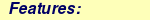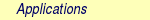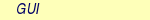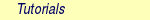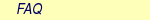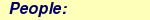MOLCAS manual:Next: 6.17 MCKINLEY Up: 6. Program Based Tutorials Previous: 6.15 Other Multiconfigurational and

Subsections

# 6.16 ALASKA and SLAPAF: A Molecular Structure Optimization

One of the most powerful functions of ab initio calculations is geometry predictions. The minimum energy structure of a molecule for a given method and basis set is instructive especially when experiment is unable to determine the actual geometry. MOLCAS performs a geometry optimization with analytical gradients at the SCF or RASSCF level of calculation, and with numerical gradients at the CASPT2 level.

In order to perform geometry optimization an input file must contain a loop, which includes several calls: calculation of integrals (SEWARD), calculation of energy (SCF, RASSCF, CASPT2), calculation of gradients (ALASKA), and calculation of the new geometry (SLAPAF).

This is an example of such input

&GATEWAY
coord= file.xyz
basis= ANO-S-MB
>> EXPORT MOLCAS_MAXITER=25
>> Do While <<
&SEWARD
&SCF
&SLAPAF
>> EndDo <<

The initial coordinates will be taken from xyz file file.xyz, and the geometry will be optimized at the SCF level in this case. After the wave function calculation, calculation of gradients is required, although code ALASKA is automatically called by MOLCAS. SLAPAF in this case required the calculation of an energy minimum (no input). Other options are transition states (TS), minimum energy paths (MEP-search), etc The loop will be terminated if the geometry converges, or maximum number of iterations (MaxIter) will be reached (the default value is 50).

There are several EMIL commands (see sect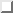) , which can be used to control geometry optimization. For example, it is possible to execute some MOLCAS modules only once:

>> IF ( ITER = 1 )
* this part of the input will be executed only during the first iteration
>> ENDIF

Program SLAPAF is tailored to use analytical or numerical gradients produced by ALASKA to relax the geometry of a molecule towards an energy minimum (default, no input required then) or a transition state. The program is also used for finding inter state crossings (ISC), conical intersections (CI), to compute reaction paths, intrinsic reaction coordinate (IRC) paths, etc.

## 6.16.1 SLAPAF - Basic and Most Common Keywords

 Keyword Meaning TS Computing a transition state FindTS Computing a transition state with a constraint MEP-search Computing a steepest-descent minimum reaction path ITER Number of iterations INTErnal Definition of the internal coordinates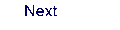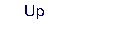Next: 6.17 MCKINLEY Up: 6. Program Based Tutorials Previous: 6.15 Other Multiconfigurational and